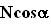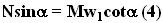## Sunday, August 12, 2007

### Irodov Problem 1.77Let N be the normal reaction between the bodies, m be the mass of the rod and M the mass of the wedge. Let us consider the forces acting on bodies one by one.

Forces acting on the rod: Since, acceleration of the rod is restricted only to the vertical direction, let us consider this direction only.There are two forces acting on the rod i) the normal reaction N it experienced from the wedge normal to the surface of contact and ii) the force of gravity mg acting downwards. Using elementary trigonometry, the component of N along the vertical direction is. Suppose that the rod's downward acceleration isthen we have,Forces acting on the wedge: The wedge moves only in the horizontal direction, and there is only one froce acting on the wedge in this direction - the component of the normal reaction from the rod onto the wedgeas shown in the figure. Let the acceleration of the wedge in the horizontal direction be. Then we have,Relation between the accelerationsand: Since the bodies are always in contact of each other, their accelerations are related.As shown in the above figure, when the rod moves from position A to A' (a distance of x units) the wedge moves from B to B' (a distance of y units). From elementary trigonometry, x and y
are related by the triangle shown in the figure. In other words,From (3) and (2)Now we can solve (1) and (4) (eliminate N) we have,From (5) and (3) we can findas,